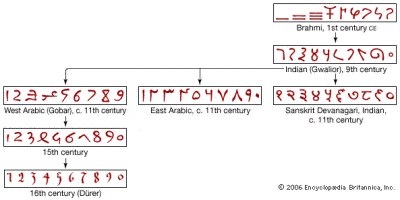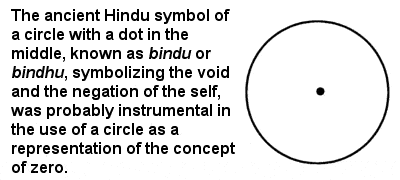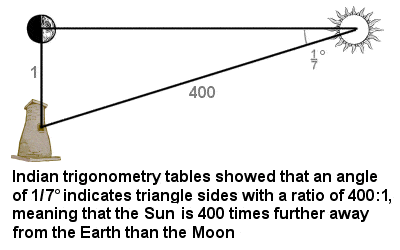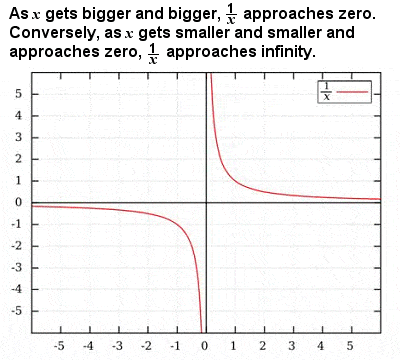# INDIAN MATHEMATICS & MATHEMATICIANSThe evolution of Hindu-Arabic numerals

Despite developing quite independently of Chinese (and probably also of Babylonian mathematics), some very advanced mathematical discoveries were made at a very early time in India.

Mantras from the early Vedic period (before 1000 BCE) invoke powers of ten from a hundred all the way up to a trillion, and provide evidence of the use of arithmetic operations such as addition, subtraction, multiplication, fractions, squares, cubes and roots. A 4th Century CE Sanskrit text reports Buddha enumerating numbers up to 1053, as well as describing six more numbering systems over and above these, leading to a number equivalent to 10421. Given that there are an estimated 1080 atoms in the whole universe, this is as close to infinity as any in the ancient world came. It also describes a series of iterations in decreasing size, in order to demonstrate the size of an atom, which comes remarkably close to the actual size of a carbon atom (about 70 trillionths of a metre).

As early as the 8th Century BCE, long before Pythagoras, a text known as the “Sulba Sutras” (or “Sulva Sutras“) listed several simple Pythagorean triples, as well as a statement of the simplified Pythagorean theorem for the sides of a square and for a rectangle (indeed, it seems quite likely that Pythagoras learned his basic geometry from the “Sulba Sutras“). The Sutras also contain geometric solutions of linear and quadratic equations in a single unknown, and give a remarkably accurate figure for the square root of 2, obtained by adding 1 + 13 + 1(3 x 4)1(3 x 4 x 34), which yields a value of 1.4142156, correct to 5 decimal places.

As early as the 3rd or 2nd Century BCE, Jain mathematicians recognized five different types of infinities: infinite in one direction, in two directions, in area, infinite everywhere and perpetually infinite. Ancient Buddhist literature also demonstrates a prescient awareness of indeterminate and infinite numbers, with numbers deemed to be of three types: countable, uncountable and infinite.

Like the Chinese, the Indians early discovered the benefits of a decimal place value number system, and were certainly using it before about the 3rd Century CE. They refined and perfected the system, particularly the written representation of the numerals, creating the ancestors of the nine numerals that (thanks to its dissemination by medieval Arabic mathematicians) we use across the world today, sometimes considered one of the greatest intellectual innovations of all time.

## Earliest Recorded Usage of a Circle Character as Number ZeroThe earliest use of a circle character for the number zero was in India

The Indians were also responsible for another hugely important development in mathematics. The earliest recorded usage of a circle character for the number zero is usually attributed to a 9th Century engraving in a temple in Gwalior in central India. But the brilliant conceptual leap to include zero as a number in its own right (rather than merely as a placeholder, a blank or empty space within a number, as it had been treated until that time) is usually credited to the 7th Century Indian mathematicians Brahmagupta – or possibly another Indian, Bhaskara I – even though it may well have been in practical use for centuries before that. The use of zero as a number which could be used in calculations and mathematical investigations, would revolutionize mathematics.

Brahmagupta established the basic mathematical rules for dealing with zero: 1 + 0 = 1; 1 – 0 = 1; and 1 x 0 = 0 (the breakthrough which would make sense of the apparently non-sensical operation 1 ÷ 0 would also fall to an Indian, the 12th Century mathematician Bhaskara II). Brahmagupta also established rules for dealing with negative numbers, and pointed out that quadratic equations could in theory have two possible solutions, one of which could be negative. He even attempted to write down these rather abstract concepts, using the initials of the names of colours to represent unknowns in his equations, one of the earliest intimations of what we now know as algebra.

The so-called Golden Age of Indian mathematics can be said to extend from the 5th to 12th Centuries, and many of its mathematical discoveries predated similar discoveries in the West by several centuries, which has led to some claims of plagiarism by later European mathematicians, at least some of whom were probably aware of the earlier Indian work. Certainly, it seems that Indian contributions to mathematics have not been given due acknowledgement until very recently in modern history.

## Indian astronomers used trigonometry tablesIndian astronomers used trigonometry tables to estimate the relative distance of the Earth to the Sun and Moon

Golden Age Indian mathematicians made fundamental advances in the theory of trigonometry, a method of linking geometry and numbers first developed by the Greeks. They used ideas like the sine, cosine and tangent functions (which relate the angles of a triangle to the relative lengths of its sides) to survey the land around them, navigate the seas and even chart the heavens.

For instance, Indian astronomers used trigonometry to calculate the relative distances between the Earth and the Moon and the Earth and the Sun. They realized that, when the Moon is half full and directly opposite the Sun, then the Sun, Moon and Earth form a right angled triangle, and were able to accurately measure the angle as 17°. Their sine tables gave a ratio for the sides of such a triangle as 400:1, indicating that the Sun is 400 times further away from the Earth than the Moon.

Although the Greeks had been able to calculate the sine function of some angles, the Indian astronomers wanted to be able to calculate the sine function of any given angle. A text called the “Surya Siddhanta”, by unknown authors and dating from around 400 CE, contains the roots of modern trigonometry, including the first real use of sines, cosines, inverse sines, tangents and secants.

As early as the 6th Century CE, the great Indian mathematician and astronomer Aryabhata produced categorical definitions of sine, cosine, versine and inverse sine, and specified complete sine and versine tables, in 3.75° intervals from 0° to 90°, to an accuracy of 4 decimal places. Aryabhata also demonstrated solutions to simultaneous quadratic equations, and produced an approximation for the value of π equivalent to 3.1416, correct to four decimal places. He used this to estimate the circumference of the Earth, arriving at a figure of 24,835 miles, only 70 miles off its true value. But, perhaps even more astonishing, he seems to have been aware that π is an irrational number, and that any calculation can only ever be an approximation, something not proved in Europe until 1761.

## Infinity as the Reciprocal of ZeroIllustration of infinity as the reciprocal of zero

Bhaskara II, who lived in the 12th Century, was one of the most accomplished of all India’s great mathematicians. He is credited with explaining the previously misunderstood operation of division by zero. He noticed that dividing one into two pieces yields a half, so 1 ÷ 12 = 2. Similarly, 1 ÷ 13 = 3. So, dividing 1 by smaller and smaller factions yields a larger and larger number of pieces. Ultimately, therefore, dividing one into pieces of zero size would yield infinitely many pieces, indicating that 1 ÷ 0 = ∞ (the symbol for infinity).

However, Bhaskara II also made important contributions to many different areas of mathematics from solutions of quadratic, cubic and quartic equations (including negative and irrational solutions) to solutions of Diophantine equations of the second order to preliminary concepts of infinitesimal calculus and mathematical analysis to spherical trigonometry and other aspects of trigonometry. Some of his findings predate similar discoveries in Europe by several centuries, and he made important contributions in terms of the systemization of (then) current knowledge and improved methods for known solutions.

The Kerala School of Astronomy and Mathematics was founded in the late 14th Century by Madhava of Sangamagrama, sometimes called the greatest mathematician-astronomer of medieval India. He developed infinite series approximations for a range of trigonometric functions, including π, sine, etc. Some of his contributions to geometry and algebra and his early forms of differentiation and integration for simple functions may have been transmitted to Europe via Jesuit missionaries, and it is possible that the later European development of calculus was influenced by his work to some extent.